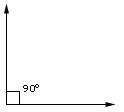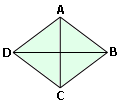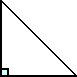## Definition Of Right Angle

Right angle is an angle that has a measure of 90°

### Examples of Right Angle

The figure below shows a right angle.### Solved Example on Right Angle

#### Ques: One of the angles in a triangle is 90° What type of a triangle is it?##### Choices:

A. equilateral triangle
B. obtuse triangle
C. right triangle
D. acute triangle